## Financial mathematics calculator, very easy to use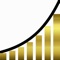# FinCalcPro Financial Solver

by Francesco Grassi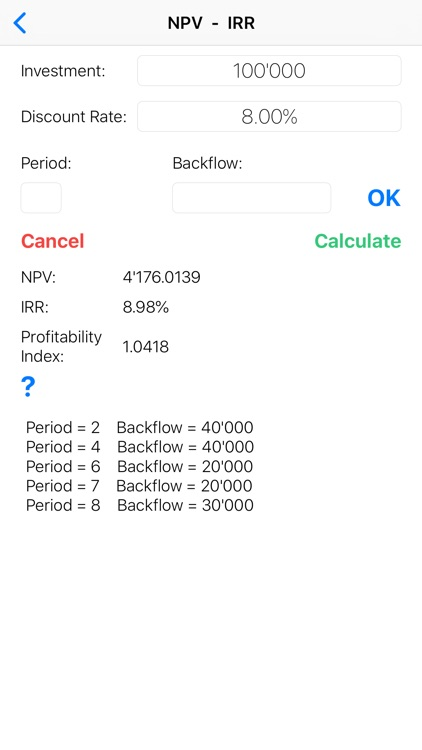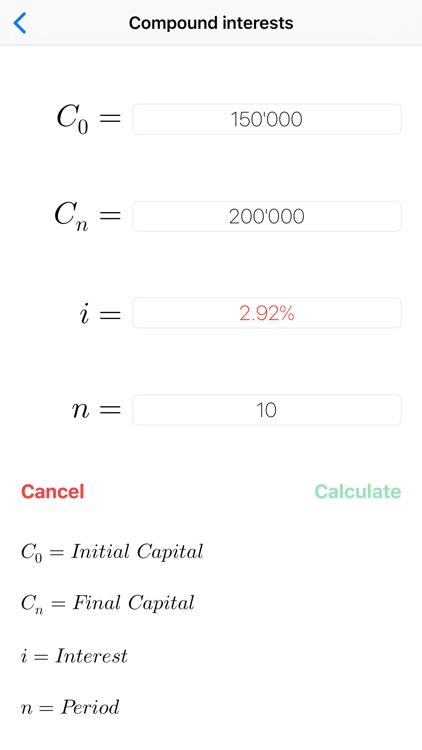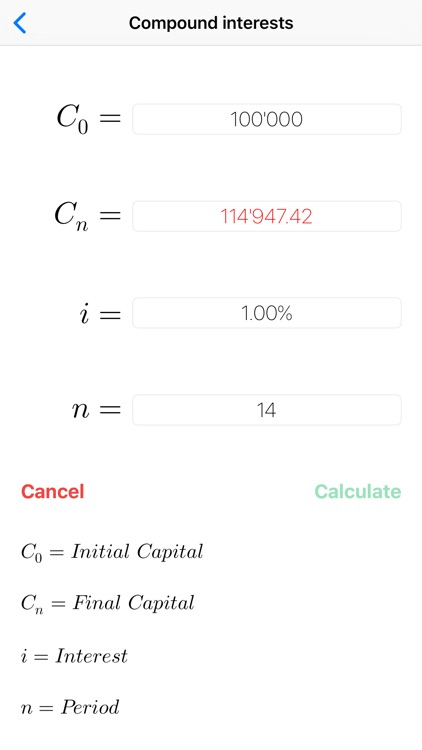Financial mathematics calculator, very easy to use.### App Details

Version
2.6
Rating
(4)
Size
3Mb
Genre
Last updated
February 18, 2021
Release date
June 18, 2015

### App Screenshots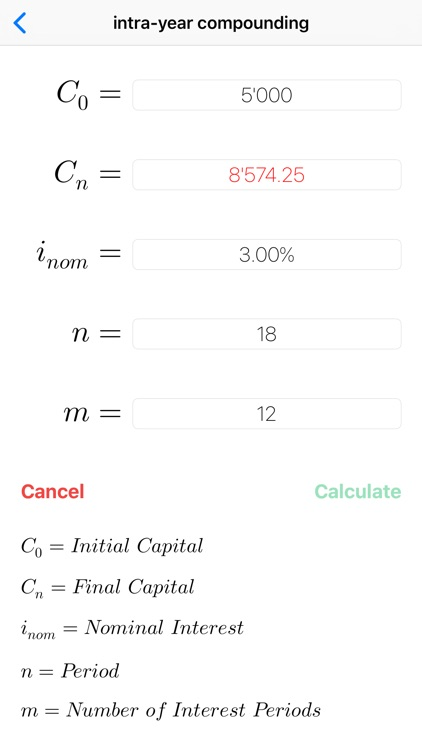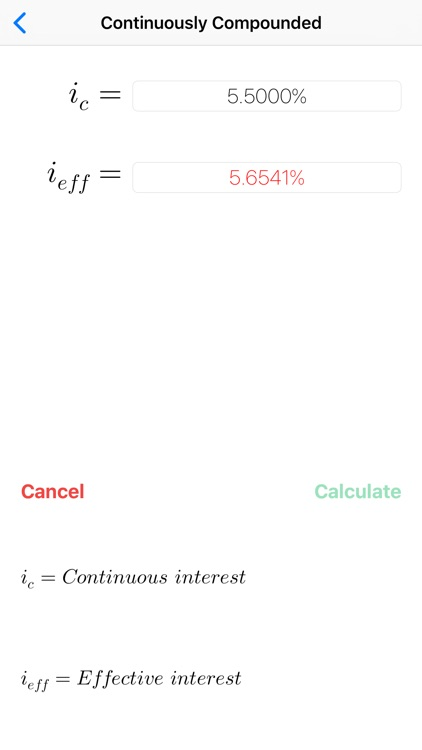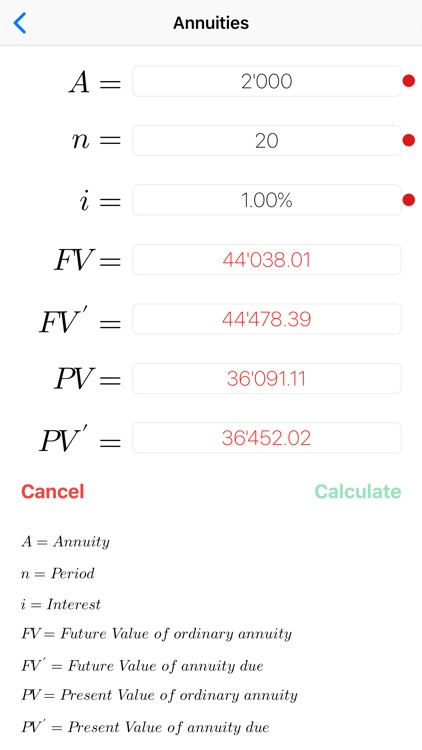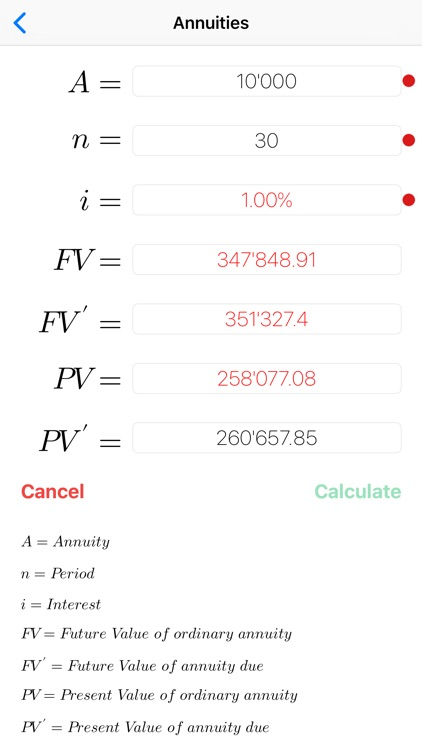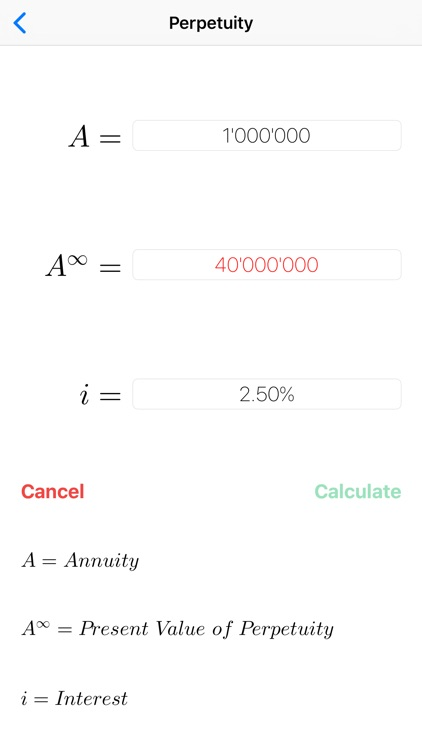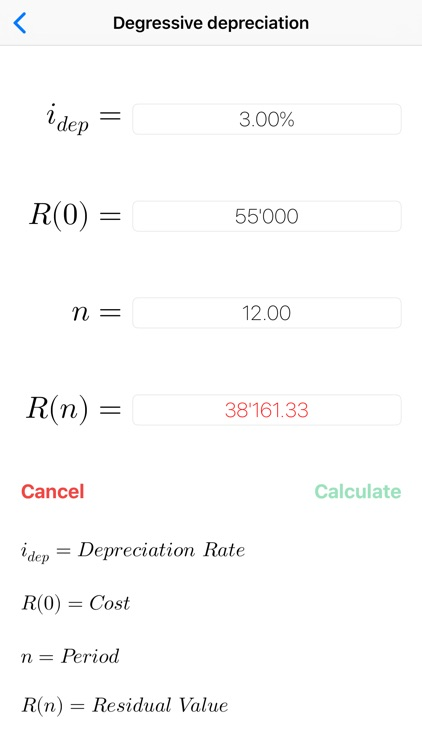### App Store Description

Financial mathematics calculator, very easy to use.
FinCalcPro CAN SOLVE EVERY VARIABLE OF THE ABOVE LIST !!!

The App can solve equations. In the screenshots the user input is black, the calculated data are red.

The following types of calculation are supported:

• PERCENTAGES
- calculation of the percentage (augmentation / reduction)
- calculation of the previous value
- calculate of the new value

• LINEAR INTEREST
- calculation of the initial capital
- calculation of the end capital
- calculation of the interest
- calculation of the period

• COMPOUND INTEREST
- calculation of the initial capital
- calculation of the end capital
- calculation of the interest
- calculation of the period

• INTRA-YEAR COMPOUNDING
- calculation of the initial capital
- calculation of the end capital
- calculation of the interest
- calculation of the period
- calculation of the number of intra-year periods
-conversion nominal interest / effective interest
- conversion effective interest / nominal interest

• CONTINUOUSLY COMPOUNDED INTEREST
- calculation of the initial capital
- calculation of the end capital
- calculation of the interest
- calculation of the period
-conversion continuous interest rate / effective interest rate
- conversion effective interest rate / continuous interest rate

• ORDINARY ANNUITIES AND ANNUITIES DUE
- calculation of the annuity
- calculation of the interest
- calculation of the final value
- calculation of the present value

• PERPETUITIES
- calculation of the annuity
- calculation of the interest
- calculation of the present value

• LINEAR DEPRECIATION
- calculation of the depreciation rate
- calculation of the new value
- calculation of the residual value

• DEGRESSIVE DEPRECIATION
- calculation of the depreciation rate
- calculation of the new value
- calculation of the residual value

• NPV - IRR
- calculation of the Net Present Value (NPV)
- calculation of the Internal Rate of Return (IRR)
- calculation of the Profitability Index

Disclaimer:
AppAdvice does not own this application and only provides images and links contained in the iTunes Search API, to help our users find the best apps to download. If you are the developer of this app and would like your information removed, please send a request to takedown@appadvice.com and your information will be removed.